# Regions Extraction of Default Mode Networks using Smith Atlas#

This simple example shows how to extract regions from Smith atlas resting state networks.

In particular, we show how Default Mode Network regions are extracted using `nilearn.regions.RegionExtractor` from regions module

Fetching the smith ICA 10 RSN by importing datasets utilities

```from nilearn import datasets

atlas_networks = datasets.fetch_atlas_smith_2009(resting=True, dimension=10)[
"maps"
]
```

Import region extractor to extract atlas networks

```from nilearn.regions import RegionExtractor

# min_region_size in voxel volume mm^3
extraction = RegionExtractor(
atlas_networks,
min_region_size=800,
threshold=98,
thresholding_strategy="percentile",
)

# Just call fit() to execute region extraction procedure
extraction.fit()
regions_img = extraction.regions_img_
```

Visualization Show region extraction results by importing image & plotting utilities

```from nilearn import plotting
from nilearn.image import index_img
from nilearn.plotting import find_xyz_cut_coords

# Showing region extraction results using 4D maps visualization tool
plotting.plot_prob_atlas(
regions_img,
display_mode="z",
cut_coords=1,
view_type="contours",
title="Regions extracted.",
)

# To reduce the complexity, we choose to display all the regions
# extracted from network 3
import numpy as np

DMN_network = index_img(atlas_networks, 3)
plotting.plot_stat_map(
DMN_network,
display_mode="z",
cut_coords=1,
title="Network 3",
colorbar=False,
)

regions_indices_network3 = np.where(np.array(extraction.index_) == 3)
for index in regions_indices_network3:
cur_img = index_img(extraction.regions_img_, index)
coords = find_xyz_cut_coords(cur_img)
plotting.plot_stat_map(
cur_img,
display_mode="z",
cut_coords=coords[2:3],
title="Blob of network3",
colorbar=False,
)

plotting.show()
```
•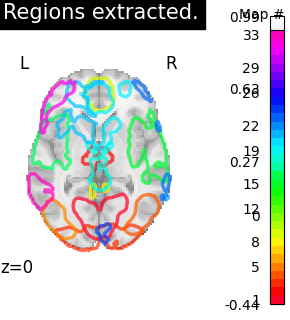•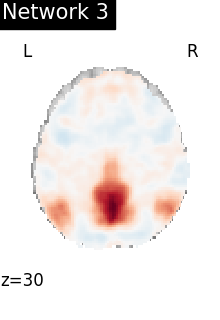•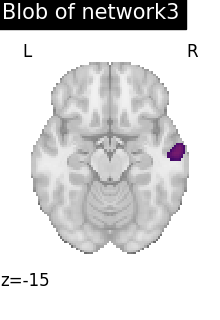•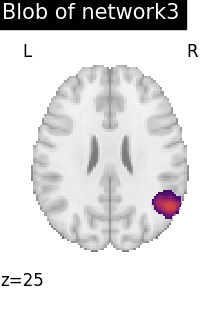•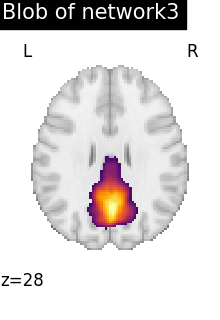•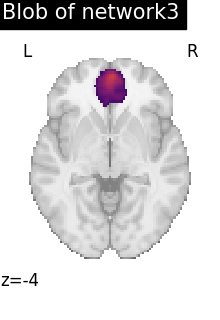•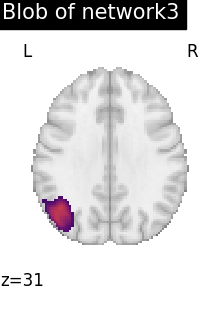•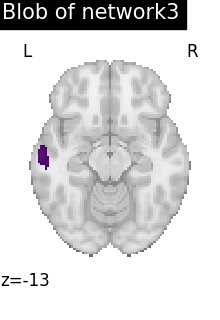Total running time of the script: (1 minutes 59.253 seconds)

Estimated memory usage: 411 MB

Gallery generated by Sphinx-Gallery